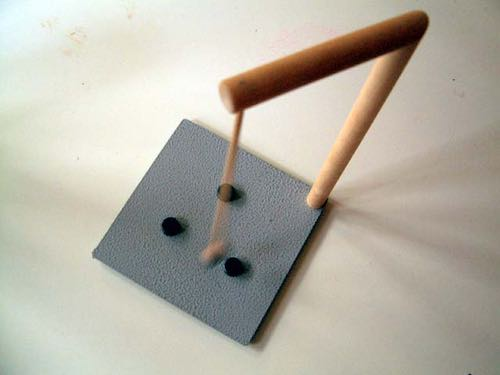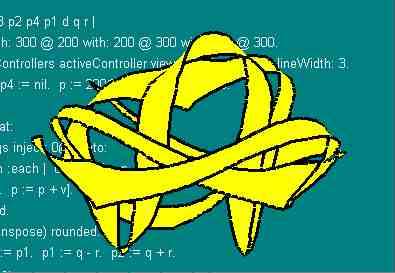#Magnetic RibbonToy magnetic pendulum.

I picked up a neat toy. It's a pendulum that swings from what looks vaguely like an eight inch high hangman's gallows. The pendulum has a magnet on the end that swings over a field of additional magnets stuck to the metal base. With two or more magnets the base the trajectory of the pendulum becomes chaotic. pageIt's a joy to watch. But after a while I wanted to understand it more. So I wrote a little pendulum simulator. I start by integrating acceleration (a) to get velocity (v), then integrating it to get position (p).

p := 200 @ 200. v := 0.01 @ 0. 4000 timesRepeat: [v := v + a. p := p + v]

Inside this loop I set acceleration to be the sum of forces from a collection of magnets (m). Each magnet (e) applies a force in direction (d) which I scale inversely by the square of the distance to the magnet.

m := Array with: 300 @ 200 with: 200 @ 300 with: 400 @ 300. a := m inject: 0@0 into: [:s :e | d := e - p. s + (d / d r squared)]Pendulum motion as ribbon with width proportional to velocity.

Now, to render the behavior of this simulation, I draw a series of polygons bounded by four vertices (p1 - p4). With each simulation step I advance the polygon by moving the leading edge (p1 p2) into the trailing edge (p3 p4) and computing a new leading edge (q +/- r) as a function of simulated position and velocity.

q := p rounded r := (10 * v transpose) rounded. p3 := p2. p4 := p1. p1 := q - r. p2 := q + r.

These polygons are rendered a GraphicsContext (gc) in yellow. Two sides of the polygon are emphasized in black to complete the effect.

gc paint: ColorValue yellow; displayPolygon: (Array with: p1 with: p2 with: p3 with: p4); paint: ColorValue black; displayLineFrom: p4 to: p1; displayLineFrom: p3 to: p2.

I run this program in VisualWorks 2.5. Interesting variations you might try include new initial conditions, more or different magnets, changing the power of the inverse square to fractional numbers like 1.8 or 2.2, and, rendering different variables or the same variables differently.

See Magnetic Morphs for Squeak version.